# NCERT Solutions for Class 9 Maths chapter 6 Exercise 6.2 - Lines and Angles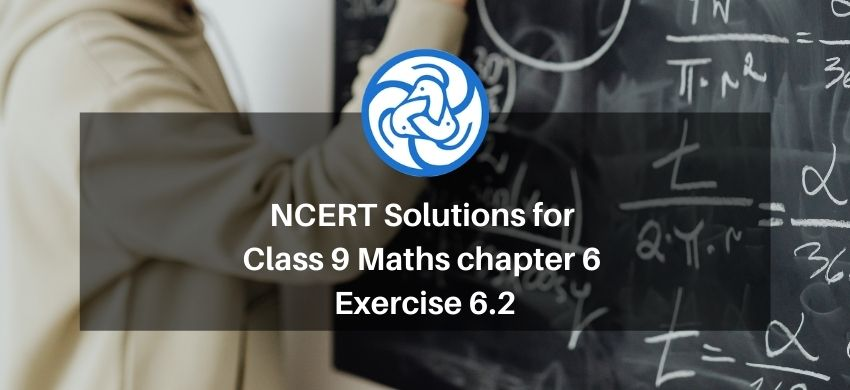Hey, are you a class 9 Student and Looking for Ways to Download NCERT Solutions for Class 9 Maths chapter 6 Exercise 6.2? If Yes then you are at the right place.

Here we have listed Class 9 maths chapter 6 exercise 6.2 solutions in PDF that is prepared by Kota’s top IITian’s Faculties by keeping Simplicity in mind.

If you want to score high in your class 9 Maths Exam then it is very important for you to have a good knowledge of all the important topics, so to learn and practice those topics you can use eSaral NCERT Solutions.

So, without wasting more time Let’s start.

### Download The PDF of NCERT Solutions for Class 9 Maths chapter 6 Exercise 6.2 "Lines and Angles"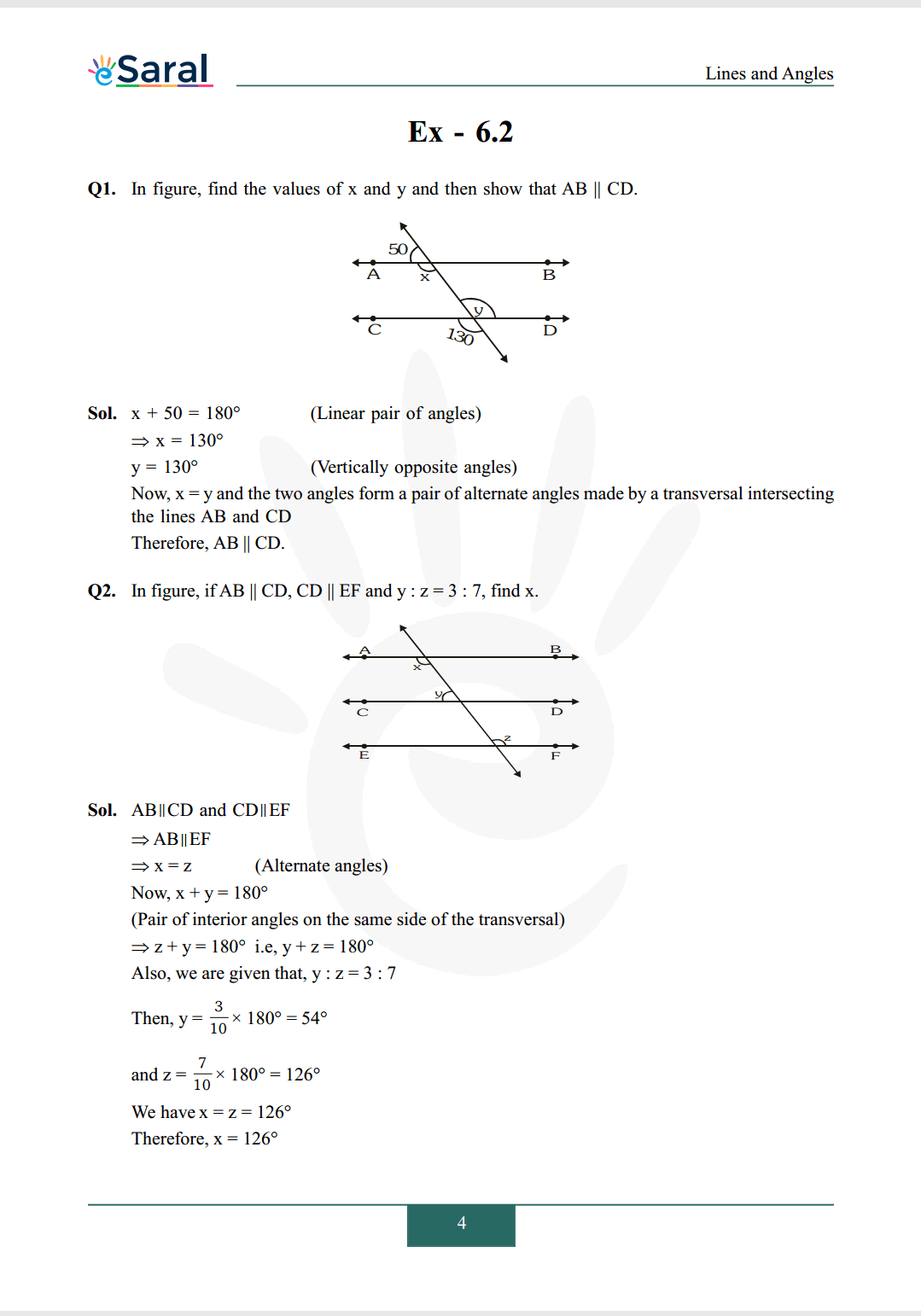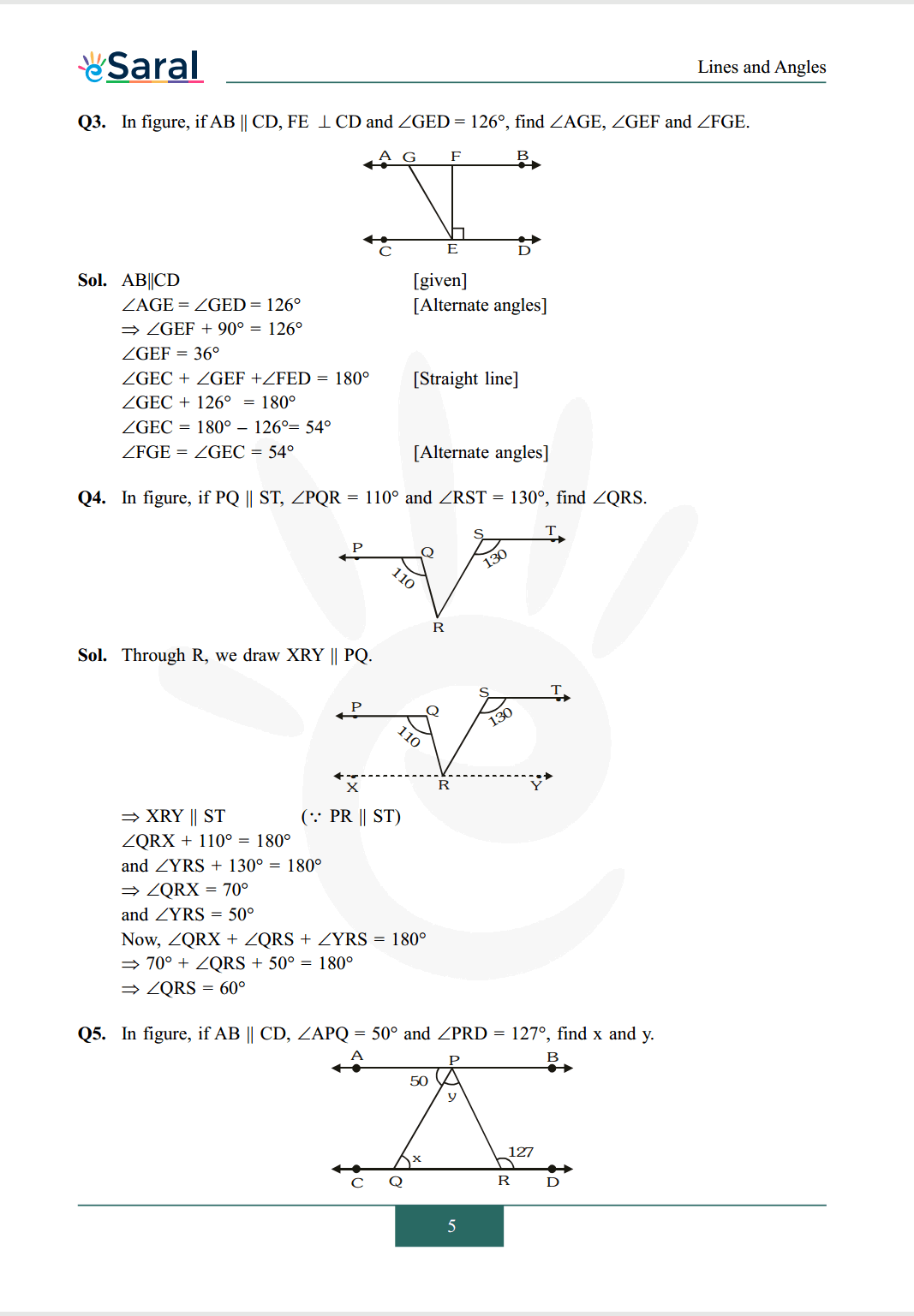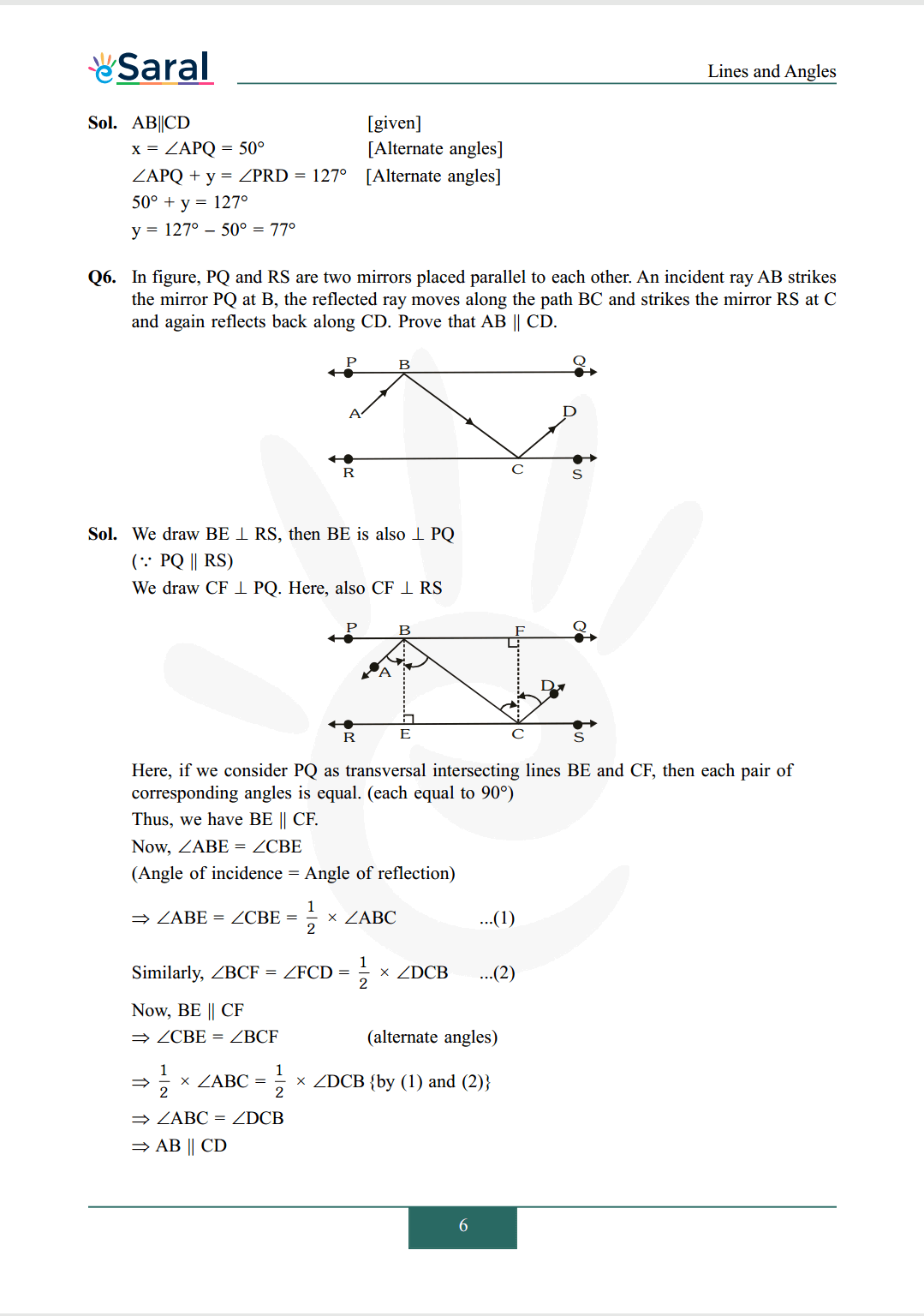#### All Questions of Chapter 6 Exercise 6.2

Once you complete the chapter 6 then you can revise Ex. 6.2 by solving following questions

Q1. In figure, find the values of $\mathrm{x}$ and $\mathrm{y}$ and then show that $\mathrm{AB} \| \mathrm{CD}$.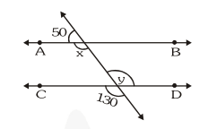Q2. In figure, if $\mathrm{AB}\|\mathrm{CD}, \mathrm{CD}\| \mathrm{EF}$ and $\mathrm{y}: \mathrm{z}=3: 7$, find $\mathrm{x}$.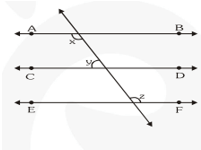Q3. In figure, if $\mathrm{AB} \| \mathrm{CD}, \mathrm{FE} \perp \mathrm{CD}$ and $\angle \mathrm{GED}=126^{\circ}$, find $\angle \mathrm{AGE}, \angle \mathrm{GEF}$ and $\angle \mathrm{FGE}$.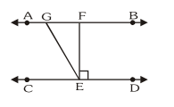Q4. In figure, if $\mathrm{PQ} \| \mathrm{ST}, \angle \mathrm{PQR}=110^{\circ}$ and $\angle \mathrm{RST}=130^{\circ}$, find $\angle \mathrm{QRS}$.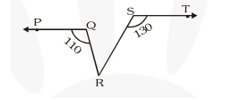Q5. In figure, if $\mathrm{AB} \| \mathrm{CD}, \angle \mathrm{APQ}=50^{\circ}$ and $\angle \mathrm{PRD}=127^{\circ}$, find $\mathrm{x}$ and $\mathrm{y}$.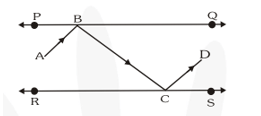Q6. In figure, $\mathrm{PQ}$ and $\mathrm{RS}$ are two mirrors placed parallel to each other. An incident ray AB strikes the mirror $\mathrm{PQ}$ at $\mathrm{B}$, the reflected ray moves along the path $\mathrm{BC}$ and strikes the mirror $\mathrm{RS}$ at $\mathrm{C}$ and again reflects back along $\mathrm{CD}$. Prove that $\mathrm{AB} \| \mathrm{CD}$.